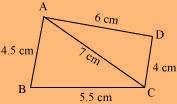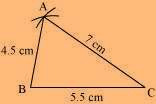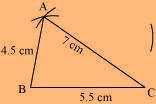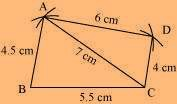# Construct the following quadrilateral: Quadrilateral ABCD AB = 4.5 cm BC = 5.5 cm CD = 4 cm AD = 6 cm AC = 7 cm

SolutionThe rough sketch of the quadrilateral ABCD can be drawn as per the steps given below

(1) ∆ABC can be constructed by using the given measurements as follows.(2) Vertex D is 6 cm away from vertex A.

Hence, while taking A as centre, draw an arc of radius 6 cm.(3) Taking C as centre, draw an arc of radius 4 cm, cutting the previous arc at point D. Joint D to A and C.